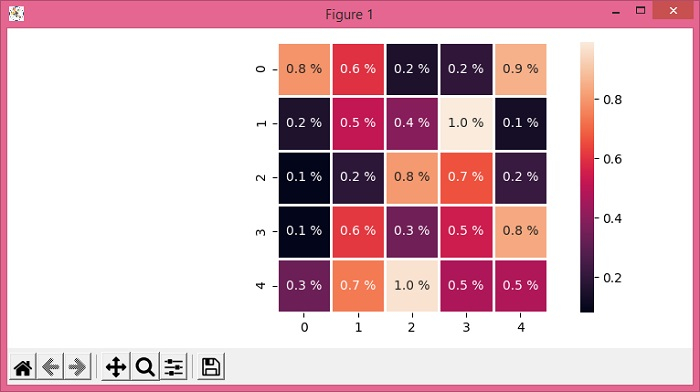# Adding units to heatmap annotation in Seaborn

To add units to a heatmap annotation in Seaborn, we can take the following steps −

• Set the figure size and adjust the padding between and around the subplots.

• Create a 5×5 dimension matrix using numpy.

• Plot rectangular data as a color-encoded matrix.

• Annotate heatmap value with %age unit.

• To display the figure, use show() method.

## Example

import matplotlib.pyplot as plt
import seaborn as sns
import numpy as np

plt.rcParams["figure.figsize"] = [7.50, 3.50]
plt.rcParams["figure.autolayout"] = True

data = np.random.rand(5, 5)

ax = sns.heatmap(data, annot=True, fmt='.1f', square=1, linewidth=1.)

for t in ax.texts: t.set_text(t.get_text() + " %")

plt.show()

## Output